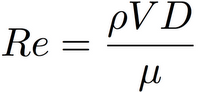# Reynold’s number

Reynolds number named after Osborne Reynolds (1842 – 1912), gives relation between inertial and viscous forces of fluid flow. If inertial forces (flow rate) are much bigger and Reynolds number is higher than critical, Re > 2320, turbulent fluid flow will occur and if viscous forces are big enough in compare to inertial (flow rate), Reynolds number is lower than critical, Re < 2320, laminar fluid flow character will occur.For calculation of Reynolds number in closed pipe fluid flow mean velocity, fluid viscosity and internal pipe diameter should be known. Reynolds number is proportional to fluid flow mean velocity and pipe diameter and inversely proportional to fluid viscosity.

Reynolds number is calculated using following equation:

The Reynolds Number is given by the formula:

#orWhere:
µ = the viscosity of the fluid,
V = the velocity of the object,
D/L = the diameter or length (basically the shape of the  object),
ρ=the density of the fluid.

With Reynolds number calculator you can analyze what makes fluid flow regime laminar and what is needed to force the fluid to flow in turbulent regime. For example – in pipes with very small internal diameter it is more likely to have laminar flow regime with small Reynolds number even for fluid with low viscosity. In fluid flow with temperature change flow regime can change from turbulent to laminar flow regime, as viscosity is changing depending on the fluid temperature.

Basic Instrumentation and Calibration